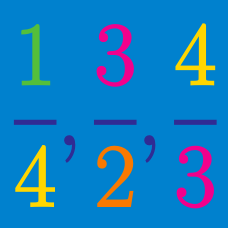Number Theory

# Rational Numbers: Level 3 Challenges

True or False?

$0.9999 \ldots = 1$


Note: The "$\ldots$" indicates that there are infinitely many 9's.

Find the number of rational numbers $r\, (0 such that when $r$ is written as a fraction in lowest terms, the numerator and denominator sum to 1000.

${A = 0.\overline{19} + 0.\overline{199}, \quad B = 0.\overline{19} \times 0.\overline{199}}$

Recall that $0.\overline{19},$ for example, stands for the repeating decimal $0.19191919...$ and that the period of a repeating decimal is the number of digits in the repeating part. In this case, the period of $0.\overline{19}$ is 2.

Find the sum of the periods of $A$ and $B$.

Let $a_k$ represent the repeating decimal $0.\overline{133}_k$ for $k \geq 4$. The product $a_4 a_5 \cdots a_{99}$ can be expressed as $\frac{m}{n!}$ where $m, n$ are positive integers and $n$ is as small as possible. $\frac{m}{n}$ can be expressed as $\frac{p}{q}$ where $p, q$ are coprime integers. What is $p+q$?

Note: $0.\overline{133}_k$ refers to the repeating decimal $0.133133133\ldots$ evalauted in base $k$.

If the following infinite series $S$ is evaluated as a decimal,what is the 37th digit to the right of the decimal place?

$\large S=\frac { 1 }{ 9 } +\frac { 1 }{ 99 } +\frac { 1 }{ 999 } +\ldots + \frac { 1 }{ { 10 }^{ n }-1 } + \ldots$

×